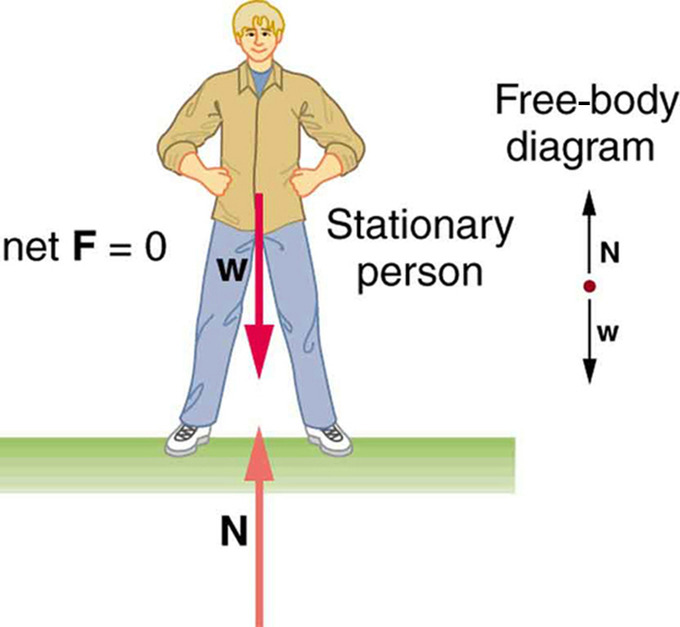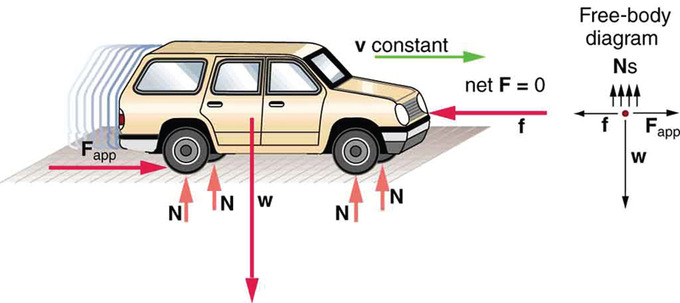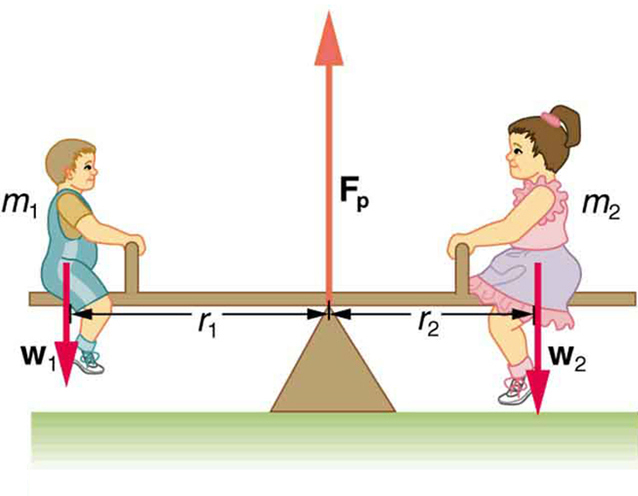First Condition

The first condition of equilibrium is that the net force in all directions must be zero.

Learning Objectives

Identify the first condition of equilibrium

Key Takeaways

Key Points

• There are two conditions that must be met for an object to be in equilibrium.
• The first condition is that the net force on the object must be zero for the object to be in equilibrium.
• If net force is zero, then net force along any direction is zero.

Key Terms

• force: A physical quantity that denotes ability to push, pull, twist or accelerate a body which is measured in a unit dimensioned in mass × distance/time² (ML/T²): SI: newton (N); CGS: dyne (dyn)
• torque: A rotational or twisting effect of a force; (SI unit newton-meter or Nm; imperial unit foot-pound or ft-lb)
• translation: Motion of a body on a linear path, without deformation or rotation, i.e. such that every part of the body moves at the same speed and in the same direction; also (in physics), the linear motion of a body considered independently of its rotation.

First Condition of Equilibrium

For an object to be in equilibrium, it must be experiencing no acceleration. This means that both the net force and the net torque on the object must be zero. Here we will discuss the first condition, that of zero net force.

In the form of an equation, this first condition is:

$\text{F}_\text{net} = 0$.

In order to achieve this conditon, the forces acting along each axis of motion must sum to zero. For example, the net external forces along the typical x– and y-axes are zero. This is written as

$\text{net } \text{F}_{\text{x}} = 0$ and $\text{net }\text{F}_{\text{y}} = 0$.

The condition $\text{F}_\text{net} = 0$ must be true for both static equilibrium, where the object’s velocity is zero, and dynamic equilibrium, where the object is moving at a constant velocity.

Below, the motionless person is in static equilibrium. The forces acting on him add up to zero. Both forces are vertical in this case.Person in Static Equilibrium: This motionless person is in static equilibrium.

Below, the car is in dynamic equilibrium because it is moving at constant velocity. There are horizontal and vertical forces, but the net external force in any direction is zero. The applied force between the tires and the road is balanced by air friction, and the weight of the car is supported by the normal forces, here shown to be equal for all four tires.A Car in Dynamic Equilibrium: This car is in dynamic equilibrium because it is moving at constant velocity. The forces in all directions are balanced.

Second Condition

The second condition of static equilibrium says that the net torque acting on the object must be zero.

Learning Objectives

Identify the second condition of static equilibrium

Key Takeaways

Key Points

• The second condition necessary to achieve equilibrium involves avoiding accelerated rotation.
• A rotating body or system can be in equilibrium if its rate of rotation is constant and remains unchanged by the forces acting on it.
• The magnitude of torque about a axis of rotation is defined to be τ=rFsinθ.

Key Terms

• torque: A rotational or twisting effect of a force; (SI unit newton-meter or Nm; imperial unit foot-pound or ft-lb)
• equilibrium: The state of a body at rest or in uniform motion, the resultant of all forces on which is zero.

A child’s seesaw, shown in, is an example of static equilibrium. An object in static equilibrium is one that has no acceleration in any direction. While there might be motion, such motion is constant.Two children on a seesaw: The system is in static equilibrium, showing no acceleration in any direction.

If a given object is in static equilibrium, both the net force and the net torque on the object must be zero. Let’s break this down:

Net Force Must Be Zero

The net force acting on the object must be zero. Therefore all forces balance in each direction. For example, a car moving along a highway at a constant speed is in equilibrium, as it is not accelerating in any forward or vertical direction. Mathematically, this is stated as Fnet = ma = 0.

Net Torque Must Be Zero

The second condition necessary to achieve equilibrium involves avoiding accelerated rotation (maintaining a constant angular velocity ). A rotating body or system can be in equilibrium if its rate of rotation is constant and remains unchanged by the forces acting on it.

To understand what factors affect rotation, let us think about what happens when you open an ordinary door by rotating it on its hinges.The magnitude, direction, and point of application of the force are incorporated into the definition of the physical quantity called torque—the rotational equivalent of a force. It is a measure of the effectiveness of a force in changing or accelerating a rotation (changing the angular velocity over a period of time).

In equation form, the magnitude of torque is defined to be τ=rFsinθ where τ (the Greek letter tau) is the symbol for torque, r is the distance from the pivot point to the point where the force is applied, F is the magnitude of the force, and θ is the angle between the force and the vector directed from the point of application to the pivot point.

Two-Component Forces

In equilibrium, the net force and torque in any particular direction equal zero.

Learning Objectives

Calculate the net force and the net torque for an object in equilibrium

Key Takeaways

Key Points

• In equilibrium, the net force in all directions is zero.
• If the net moment of inertia about an axis is zero, the object will have no rotational acceleration about the axis.
• In each direction, the net force takes the form: $\sum \textbf{F}=\text{m}\textbf{a}=0$ and the net torque take the form: $\sum \boldsymbol{\tau}=\text{I}\boldsymbol{\alpha}=0$ where the sum represents the vector sum of all forces and torques acting.

Key Terms

• equilibrium: The state of a body at rest or in uniform motion, the resultant of all forces on which is zero.

An object with constant velocity has zero acceleration. A motionless object still has constant (zero) velocity, so motionless objects also have zero acceleration. Newton’s second law states that:

$\sum \textbf{F}=\text{m}\textbf{a}$

so objects with constant velocity also have zero net external force. This means that all the forces acting on the object are balanced — that is to say, they are in equilibrium.

This rule also applies to motion in a specific direction. Consider an object moving along the x-axis. If no net force is applied to the object along the x-axis, it will continue to move along the x-axis at a constant velocity, with no acceleration.Car Moving at Constant Velocity: A moving car for which the net x and y force components are zero

We can easily extend this rule to the y-axis. In any system, unless the applied forces cancel each other out (i.e., the resultant force is zero), there will be acceleration in the direction of the resultant force. In static systems, in which motion does not occur, the sum of the forces in all directions always equals zero. This concept can be represented mathematically with the following equations:

$\sum \text{F}_{\text{x}}=\text{ma}_{\text{x}}=0$

$\sum \text{F}_{\text{y}}=\text{ma}_{\text{y}}=0$

This rule also applies to rotational motion. If the resultant moment about a particular axis is zero, the object will have no rotational acceleration about the axis. If the object is not spinning, it will not start to spin. If the object is spinning, it will continue to spin at the same constant angular velocity. Again, we can extend this to moments about the y-axis as well. We can represent this rule mathematically with the following equations:

$\sum \tau_{\text{x}}=\text{I}\alpha_{\text{x}}=0$

$\sum \tau_{\text{y}}=\text{I}\alpha_{\text{y}}=0$